# The ARIMA Procedure

### Specifying Inputs and Transfer Functions

Subsections:

Input variables and transfer functions for them can be specified using the INPUT= option in the ESTIMATE statement. The variables used in the INPUT= option must be included in the CROSSCORR= list in the previous IDENTIFY statement. If any differencing is specified in the CROSSCORR= list, then the differenced variable is used as the input to the transfer function.

#### General Syntax of the INPUT= Option

The general syntax of the INPUT= option is

• ESTIMATE …INPUT=( transfer-function variable …)

The transfer function for an input variable is optional. The name of a variable by itself can be used to specify a pure regression term for the variable.

If specified, the syntax of the transfer function is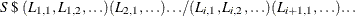S is the number of periods of time delay (lag) for this input series. Each term in parentheses specifies a polynomial factor with parameters at the lags specified by the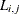values. The terms before the slash (/) are numerator factors. The terms after the slash (/) are denominator factors. All three parts are optional.

Commas can optionally be used between input specifications to make the INPUT= option more readable. The \$ sign after the shift is also optional.

Except for the first numerator factor, each of the terms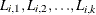indicates a factor of the form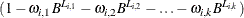The form of the first numerator factor depends on the ALTPARM option. By default, the constant 1 in the first numerator factor is replaced with a free parameter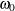.

#### Alternative Model Parameterization

When the ALTPARM option is specified, theparameter is factored out so that it multiplies the entire transfer function, and the first numerator factor has the same form as the other factors.

The ALTPARM option does not materially affect the results; it just presents the results differently. Some people prefer to see the model written one way, while others prefer the alternative representation. Table 7.9 illustrates the effect of the ALTPARM option.

Table 7.9: The ALTPARM Option

 INPUT= Option ALTPARM Model INPUT=((1 2)(12)/(1)X); No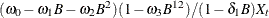Yes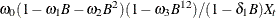#### Differencing and Input Variables

If you difference the response series and use input variables, take care that the differencing operations do not change the meaning of the model. For example, if you want to fit the model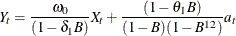then the IDENTIFY statement must read

   identify var=y(1,12) crosscorr=x(1,12);
estimate q=1 input=(/(1)x) noconstant;


If instead you specify the differencing as

   identify var=y(1,12) crosscorr=x;
estimate q=1 input=(/(1)x) noconstant;


then the model being requested is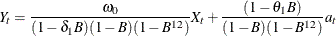which is a very different model.

The point to remember is that a differencing operation requested for the response variable specified by the VAR= option is applied only to that variable and not to the noise term of the model.